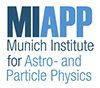#Exploring the Perfect Liquid

Sep 6 – 8, 2018
Max Planck Institute for Astrophysics (MPA)
Europe/Berlin timezone

## Non-Bessel-Gaussianity and Flow Harmonic Fine-Splitting

Sep 8, 2018, 12:25 PM
20m
E.0.11 (Max Planck Institute for Astrophysics (MPA))

### E.0.11

#### Max Planck Institute for Astrophysics (MPA)

Karl-Schwarzschild-Str. 1 85748 Garching
Flow

### Speaker

Dr Seyed Farid Taghavi (school of particles and accelerators, institute for research in fundamental sciences, Tehran, Iran)

### Description

Both collision geometry and event-by-event fluctuations are encoded in the experimentally observed flow harmonic distribution $p(v_n)$ and 2k-particle cumulants $c_n\{2k\}$. In the present talk, we systematically connect these observables to each other by employing Gram-Charlier A series. Also we quantify the deviation of the flow harmonic distribution from Bessel-Gaussianity in terms of the flow harmonic fine-splitting. This study helps us to disentangle the effect of the collision geometry and fluctuations in $v_n\{2k\}$. After that we introduce two applications for this study. We first introduce several estimators for the averaged ellipticity and second we restrict the $v_2\{2\}$, $v_2\{4\}$ and $v_2\{8\}$ phase space.

### Primary author

Dr Seyed Farid Taghavi (school of particles and accelerators, institute for research in fundamental sciences, Tehran, Iran)